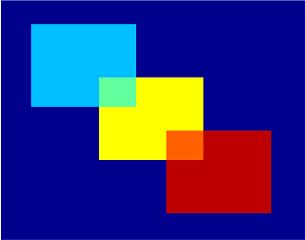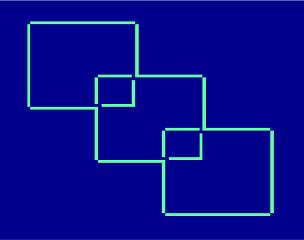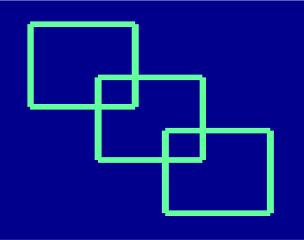# Line detection on an image

This experiment explores line detection (for image analysis) with Matlab.
 Since an image can be expressed with two matrices (one matrix being the color palette and the other one being the reference to those colors), we can manipulate pictures or images by modifying both the map (palette) and the matrix containing the values to be displayed.

This experiment draws some squares in different colors. These are the coded details:

% We choose the 'jet' palette
colormap jet

% We define a 90 x 90 matrix, and make it all ones.
% This means that the 'background' will be the first
%    element found in our palette

r = 90; c = r;
W = ones(r, c);

% Define our colors in very specific areas of the matrix
W(10 : 40, 10 : 40) = 20;
W(30 : 60, 30 : 60) = 40;
W(50 : 80, 50 : 80) = 60;
W(30 : 40, 30 : 40) = 30;
W(50 : 60, 50 : 60) = 50;

% Display the image and 'turn off' the axes
image(W); axis off
save color_squares W

This is the resulting image or picture:Now, we’ll inspect the matrix pixel-by-pixel (a pixel is a picture element). If we find different colors between two consecutive pixels, we’ll write it down on another matrix that finally will be shown.

We can have different approaches to the inpection of consecutive pixels.
We can do these operations, for example (if i and j represent rows and columns of the matrix):

(W(i-1, j) - W(i,j)) + (W(i+1, j) - W(i,j)) +...
(W(i, j-1) - W(i,j)) + (W(i, j+1) - W(i,j));

or

(W(i-1, j) - W(i,j))^2 + (W(i+1, j) - W(i,j))^2 +...
(W(i, j-1) - W(i,j))^2 + (W(i, j+1) - W(i,j))^2;

If the result is greater than 0 it means that we’ve found different objects (or edges) within a picture and we can annotate that information on another matrix, to be displayed later.

This is the full code to the first approach:

[r, c] = size(W);
W2 = ones(r, c);

for i = 2 : r-1

for j = 2 : c-1
cl(i, j) = (W(i-1, j) - W(i,j)) + (W(i+1, j) - W(i,j)) +
...
(W(i, j-1) - W(i,j)) + (W(i, j+1) - W(i,j));

if cl(i,j) > 0
W2(i,j) = 30;

end
end
end

image(W2); axis off

It produces this image:We can change some lines in the code to achieve our second approach:

cl(i, j) = (W(i-1, j) - W(i,j))^2 + (W(i+1, j) - W(i,j))^2 +...
(W(i, j-1) - W(i,j))^2 + (W(i, j+1) - W(i,j))^2;

which produces this picture:and so we have performed an edge or line detection from our original image under study.

From 'Line Detection' to home

From 'Line Detection' to 'Matlab Programming'